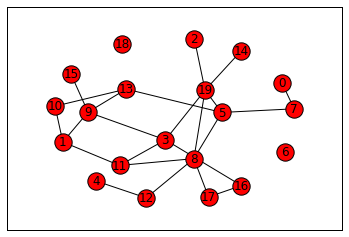Once again, I was playing around with Jupyter. This time I was more interested in seeing how it handled NetworkX. It seemed to work fairly well, though the kernel died using above 500 connected nodes.

# Networks

## Create a Erdős–Rényi random graph

``````import networkx as nx
g = nx.erdos_renyi_graph(20,0.10)
``````

## Plot the graph

``````import matplotlib.pyplot as plt

fig, ax = plt.subplots()
# disable some extras we don't want
ax.grid(False)
ax.xaxis.set_visible(False)
ax.yaxis.set_visible(False)
# draw the graph
nx.draw_networkx(g,ax=ax)
``````Tags: Jupyter
Part of a series on Jupyter.